#### Application of Eigenvectors-Vibrational Modes

An Application of Eigenvectors:

Vibrational Modes:

One application of ew’s as well as ev’s is in the analysis of vibration problems. A easy nontrivial vibration problem is the motion of two objects with equal masses m attached to each other and fixed outer walls by equal springs with spring constants k.

Let x1 signify the displacement of the first mass and x2 the displacement of the second and note the displacement of the walls is zero. Every mass experiences forces from the adjacent springs proportional to the stretch or compression of the spring. Disregard any friction Newton’s law of motion ma = F, leads to:

m¨x1 = −k(x1− 0) +k(x2− x1) = −2kx1 + kx2
m¨x2 = −k(x2− x1) +k(0 − x2) = kx1− 2kx2

Dividing both sides by m we are able to write these equations in matrix form:

¨x = −Ax,

Where,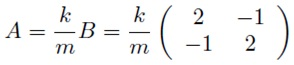For this kind of equation the general solution is: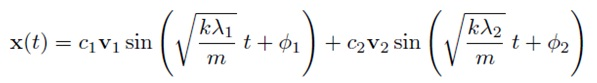Where λ1 as well as λ2 are ew’s of B with corresponding ev’s v1 and v2. One can ensure that this is a solution by substituting it into the equation.

We are able to interpret the ew’s as the squares of the frequencies of oscillation. We can locate the ew’s and ev’s of B using Mat lab

> B = [2 -1 ; -1 2]
> [v e] = eig(B)

This must produce a matrix v whose columns are the ev’s of B and a diagonal matrix e whose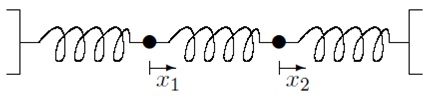Two equivalent masses attached to each other and fixed walls by equal springs.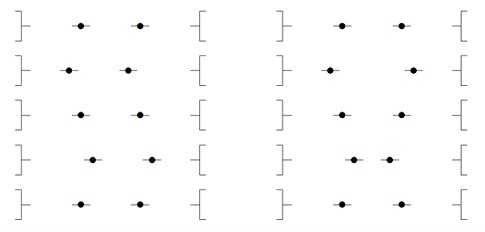Two vibrational modes of a easy oscillating system. In the left mode the weights move together as well as in the right mode they move opposite. Note that the two modes in fact move at different speeds.

Entries are the ew’s of B In the primary eigenvector v1 the two entries are equal. This stands for the mode of oscillation where the two masses move in sync with each other. The second ev, v2 has the similar entries but opposite signs. This signifies the mode where the two masses oscillate in anti-synchronization.

Notice that the occurrence for anti-sync motion is √3 times that of synchronous motion.

Which of the two modes is the largely dangerous for a structure or machine? It is the one with the lowest frequency for the reason that that mode can have the largest displacement. Occasionally this mode is called the fundamental mode.

To obtain the frequencies for the matrix A = k/mB notice that if viis one of the ev’s for B then: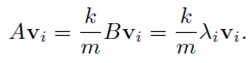Therefore we can conclude that A has the same ev’s as B but the ew’s are multiplied by the factor k/m. Therefore the two frequencies are: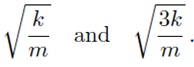We are able to do the same for three equal masses the corresponding matrix B would be: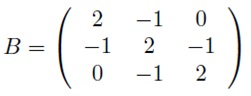Find the ev’s as well as ew’s as above. There are three dissimilar modes Interpret them from the ev’s.

Latest technology based Matlab Programming Online Tutoring Assistance

Tutors, at the www.tutorsglobe.com, take pledge to provide full satisfaction and assurance in Matlab Programming help via online tutoring. Students are getting 100% satisfaction by online tutors across the globe. Here you can get homework help for Matlab Programming, project ideas and tutorials. We provide email based Matlab Programming help. You can join us to ask queries 24x7 with live, experienced and qualified online tutors specialized in Matlab Programming. Through Online Tutoring, you would be able to complete your homework or assignments at your home. Tutors at the TutorsGlobe are committed to provide the best quality online tutoring assistance for Matlab Programming Homework help and assignment help services. They use their experience, as they have solved thousands of the Matlab Programming assignments, which may help you to solve your complex issues of Matlab Programming. TutorsGlobe assure for the best quality compliance to your homework. Compromise with quality is not in our dictionary. If we feel that we are not able to provide the homework help as per the deadline or given instruction by the student, we refund the money of the student without any delay.